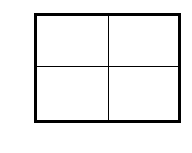Home > CCA > Chapter Ch4 > Lesson 4.2.3 > Problem4-64

4-64.

Use generic rectangles to multiply each of the following expressions. Homework Help ✎

1. $( x + 2 ) ( x - 5 )$

• Draw a generic rectangle.

• Multiply the value of the column and the value of the row to find the value of each box in the rectangle.

• $(x+2)(x−5)=x^2−3x−10$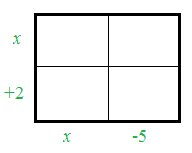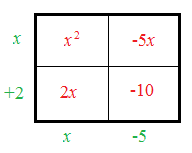1. $( y + 2 x ) ( y + 3 x )$

• See the hints in part (a).

• $(y+2x)(y+3x)=y^2+5xy+6x^2$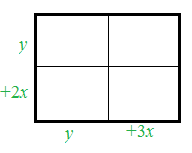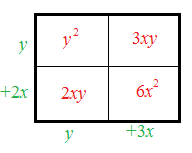1. $( 3 y - 8 ) ( - x + y )$

• See the hints in parts (a) and (b).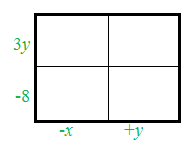1. $(x-3y)(x+3y)$

• See the hints in part (a).